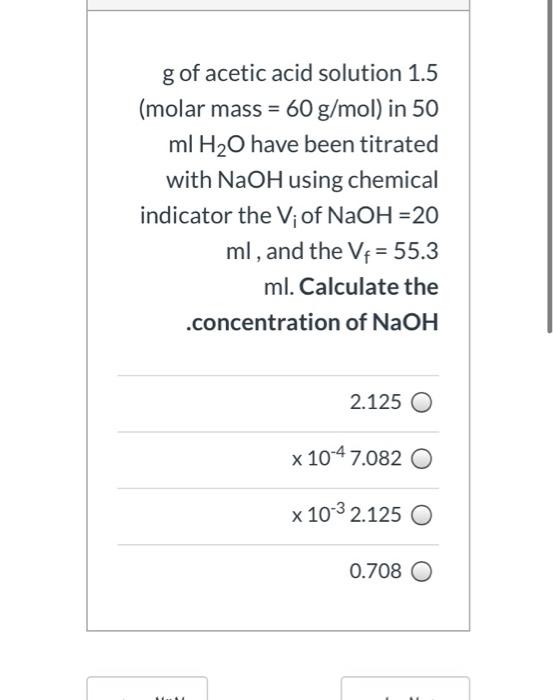### Create an Account

Home / Questions / g of acetic acid solution 1.5 (molar mass = 60 g/mol) in 50 ml H2O have been titrated with...

# g of acetic acid solution 1.5 (molar mass = 60 g/mol) in 50 ml H2O have been titrated with NaOH using chemical indicator the V; of NaOH =20 ml, and the V+ = 55.3 ml. Calculate the .concentration of

g of acetic acid solution 1.5 (molar mass = 60 g/mol) in 50 ml H2O have been titrated with NaOH using chemical indicator the V; of NaOH =20 ml, and the V+ = 55.3 ml. Calculate the .concentration of NaOH 2.125 x 10-4 7.082 0 x 10-3 2.125 O 0.708May 18 2021 View more View LessSubscribe To Get Solution